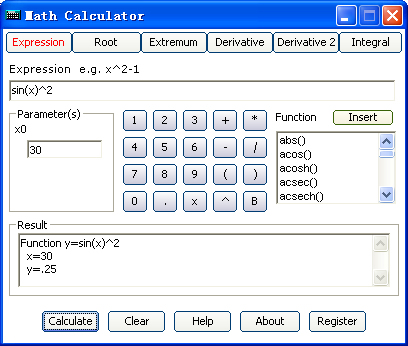Math Calculator
 Advertisement

# Math Calculator ScreenshotsScreenshots for Math Calculator

Math Calculator Review
 Abilities of expression calculating, solving equation, finding extremum, derivative calculating and integral calculating. ...[Read More]

Sponsored Links

 POPULAR LINKS